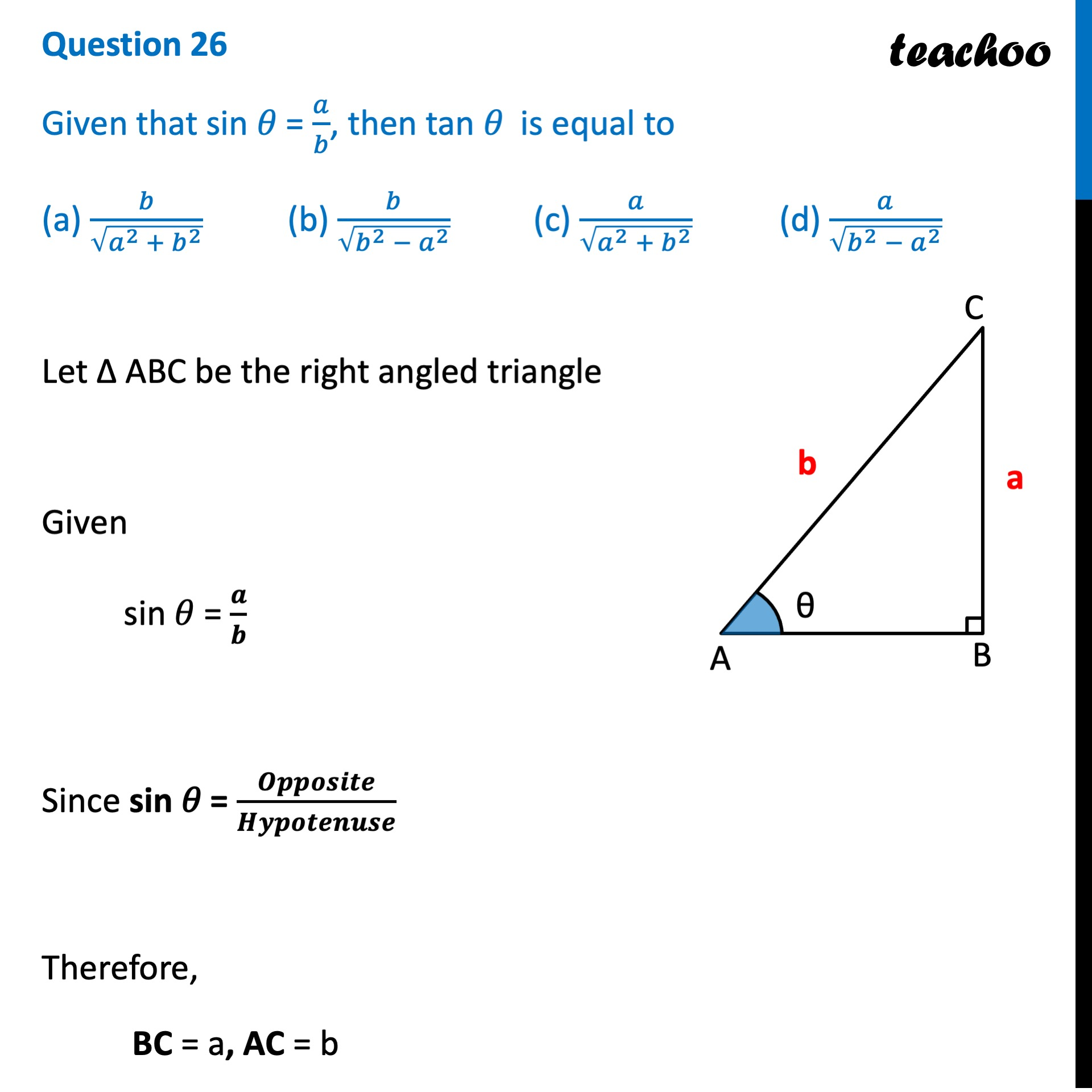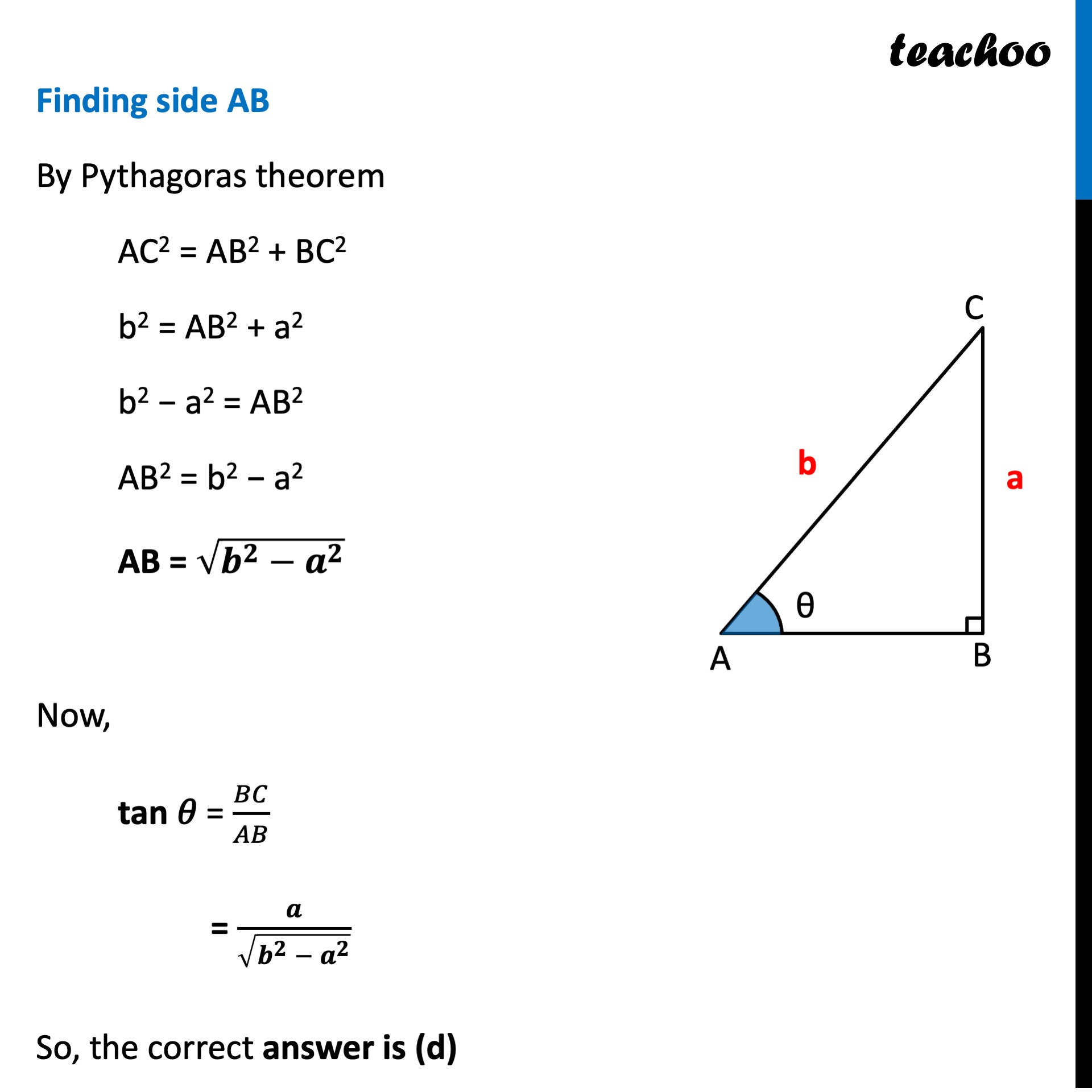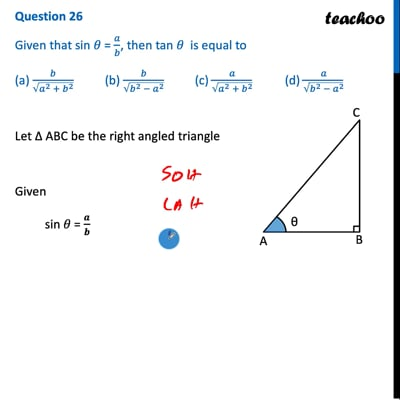CBSE Class 10 Sample Paper for 2022 Boards - Maths Basic [MCQ]

Class 10
Solutions of Sample Papers for Class 10 Boards

##(adsbygoogle = window.adsbygoogle || []).push({});(adsbygoogle = window.adsbygoogle || []).push({});This video is only available for Teachoo black users

### Transcript

Question 26 Given that sin 𝜃 = 𝑎/𝑏, then tan 𝜃 is equal to (a) 𝑏/√(𝑎^2 + 𝑏^2 ) (b) 𝑏/√(𝑏^2 − 𝑎^2 ) (c) 𝑎/√(𝑎^2 + 𝑏^2 ) (d) 𝑏/√(𝑏^2 + 𝑎^2 ) Let Δ ABC be the right angled triangle Given sin 𝜃 = 𝒂/𝒃 Since sin 𝜃 = 𝑶𝒑𝒑𝒐𝒔𝒊𝒕𝒆/𝑯𝒚𝒑𝒐𝒕𝒆𝒏𝒖𝒔𝒆 Therefore, BC = a, AC = b Finding side AB By Pythagoras theorem AC2 = AB2 + BC2 b2 = AB2 + a2 b2 − a2 = AB2 AB2 = b2 − a2 AB = √(𝒃^𝟐−𝒂^𝟐 ) Now, tan 𝜃 = 𝐵𝐶/𝐴𝐵 = 𝒂/√(𝒃^𝟐 − 𝒂^𝟐 ) So, the correct answer is (d)